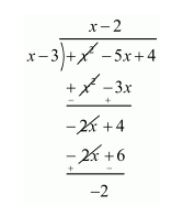# What should be added to the polynomial x2 − 5x + 4,`
Question:

What should be added to the polynomial $x^{2}-5 x+4$, so that 3 is the zero of the resulting polynomial?

(a) 1
(b) 2
(c) 4
(d) 5

Solution:

If $x=\alpha$, is a zero of a polynomial then $x-\alpha$ is a factor of $f(x)$

Since 3 is the zero of the polynomial $f(x)=x^{2}-5 x+4$,

Therefore $x-3$ is a factor of $f(x)$

Now, we divide $f(x)=x^{2}-5 x+4$ by $(x-3)$ we getTherefore we should add 2 to the given polynomial

Hence, the correct choice is $(b)$